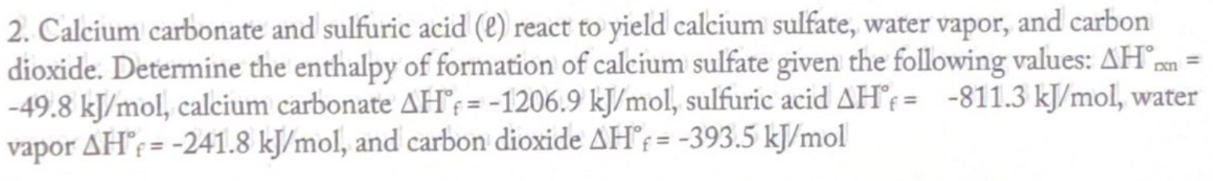# 2. Calcium carbonate and sulfuric acid (l) react to yield calcium sulfate, water vapor, and carbon dioxide. Determine the enthalpy of formation of calcium sulfate given the following values: ΔH° rxn = -49.8 kJ/mol, calcium carbonate ΔH°f= -1206.9 kJ/mol, sulfuric acid ΔH°f = -811.3 kJ/mol, water vapor ΔH°f = -241.8 kJ/mol, and carbon dioxide ΔH° f = -393.5 kJ/mol